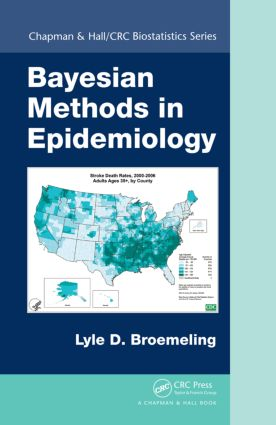# Bayesian Methods in Epidemiology

## 1st Edition

Chapman and Hall/CRC

464 pages | 59 B/W Illus.

##### Purchasing Options:\$ = USD
Hardback: 9781466564978
pub: 2013-08-13
SAVE ~\$21.00
\$105.00
\$84.00
x
eBook (VitalSource) : 9780429195051
pub: 2013-08-13
from \$49.48

FREE Standard Shipping!

### Description

Written by a biostatistics expert with over 20 years of experience in the field, Bayesian Methods in Epidemiology presents statistical methods used in epidemiology from a Bayesian viewpoint. It employs the software package WinBUGS to carry out the analyses and offers the code in the text and for download online.

The book examines study designs that investigate the association between exposure to risk factors and the occurrence of disease. It covers introductory adjustment techniques to compare mortality between states and regression methods to study the association between various risk factors and disease, including logistic regression, simple and multiple linear regression, categorical/ordinal regression, and nonlinear models. The text also introduces a Bayesian approach for the estimation of survival by life tables and illustrates other approaches to estimate survival, including a parametric model based on the Weibull distribution and the Cox proportional hazards (nonparametric) model. Using Bayesian methods to estimate the lead time of the modality, the author explains how to screen for a disease among individuals that do not exhibit any symptoms of the disease.

With many examples and end-of-chapter exercises, this book is the first to introduce epidemiology from a Bayesian perspective. It shows epidemiologists how these Bayesian models and techniques are useful in studying the association between disease and exposure to risk factors.

### Reviews

"… the only book that I am aware of in literature that introduces epidemiology from a Bayesian point of view. … Each concept has been introduced in its standard definition as well as its analogy in the Bayesian approach, thereby making it easier for non-Bayesian researchers to understand. The book is very well written and structured in a way that makes the comprehension of the introduced concepts very smooth. The comprehensive range of covered topics is impressive but not daunting. … a great textbook for any researcher with considerable statistical background who is interested in applying Bayesian methods to public health data. … useful as a refresher course … I would highly recommend Bayesian Methods in Epidemiology as an introductory text to the subject as a whole. … also a valuable resource for experienced Bayesian analysts."

Statistics in Medicine, 34, 2015

"This is a useful and interesting book … This book will help initiate those unfamiliar with Bayesian principles in Bayesian thinking and further implement Bayesian methodology in solving problems of import in medical applications. … an exciting book, which I believe has the potential to help public health practitioners identify important risk factors for disease and make important policy decisions."

International Statistical Review, 82, 2014

"This book consists of some examples of Bayesian methods applications to the analysis of epidemiological statistical data. It covers analysis of associations between risk exposures and diseases; adjustment of mortality data; regression methods; life tables analysis; survival analysis with Bayesian Kaplan-Meyer methods and Cox proportional hazards models; and screening for diseases."

—R.E. Maiboroda, Zentralblatt MATH

"…by reading the book and working through the author’s examples, and the examples at the end of each chapter, analysts working in epidemiology are supposed to gain an understanding of how Bayesian methods might be applied to their own work."

The American Statistician

"In Bayesian Methods in Epidemiology, Dr. Broemeling brings his vast working expe-rience in the ?eld to a practitioner’s guide for epidemiologic principles solved the Bayesian way. Illustrations with real data from important studies, a host of exercises, politely sub-tle references to appendices for review, and an abundance of WinBUGS code make this book highly accessible to scientists working across the quantitative spectrum."

—Biometrics

### Table of Contents

Introduction to Bayesian Methods in Epidemiology

Introduction

Review of Statistical Methods in Epidemiology

Preview of the Book

Preview of the Appendices

A Bayesian Perspective of Association between Risk Exposure and Disease

Introduction

Incidence and Prevalence for Mortality and Morbidity

Association between Risk and Disease in Cohort Studies

Retrospective Studies: Association between Risk and Disease in Case–Control Studies

Cross-Sectional Studies

Attributable Risk

Bayesian Methods of Adjustment of Data

Introduction

The Direct Adjustment of Data

Indirect Standardization Adjustment

Stratification and Association between Disease and Risk Exposure

Mantel–Haenszel Estimator of Association

Matching to Adjust Data in Case–Control Studies

Regression Methods for Adjustment

Introduction

Logistic Regression

Linear Regression Models

Weighted Regression

Ordinal and Other Regression Models

A Bayesian Approach to Life Tables

Introduction

The Basic Life Table

Disease-Specific Life Tables

Life Tables for Medical Studies

Comparing Survival

The Kaplan–Meier Test

A Bayesian Approach to Survival Analysis

Introduction

Notation and Basic Table for Survival

Kaplan–Meier Survival Curves

Survival Analysis

Screening for Disease

Introduction

Principals of Screening

Evaluation of Screening Programs

The HIP Study (Health Insurance Plan of Greater New York)

Statistical Models for Epidemiology

Introduction

Review of Models for Epidemiology

Categorical Regression Models

Nonlinear Regression Models

Repeated Measures Model

Spatial Models for Epidemiology

Appendix A: Introduction to Bayesian Statistics

Appendix B: Introduction to WinBUGS

Index

Comments, Conclusions, Exercises, and References appear at the end of each chapter.

### Subject Categories

##### BISAC Subject Codes/Headings:
MAT029000
MATHEMATICS / Probability & Statistics / General
MED028000
MEDICAL / Epidemiology
MED090000
MEDICAL / Biostatistics